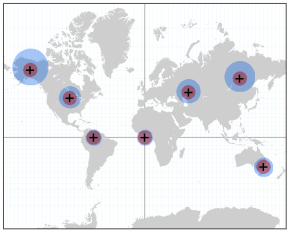# Geodesic versus planar distance

The distance tools include an option to perform calculations using planar distance or geodesic distance. Planar distance is straight-line Euclidean distance calculated in a 2D Cartesian coordinate system. Geodesic distance is calculated in a 3D spherical space as the distance across the curved surface of the world.

## Distance analysis and map projections

The earth is a three-dimensional slightly flattened sphere, or ellipsoid. To represent the earth on maps, cartographers created map projections, which are mathematical transformations between 3D and 2D space. These projections distort the data in different ways, affecting measurement of distances, angles, and areas. Many projections have been developed in attempts to preserve one or more of these characteristics, often for particular parts of the map such as specific meridians or parallels, or a few points. Although some map projections preserve some characteristics accurately, none preserve all distances correctly. Projections have been developed that preserve true direction, but none fully preserve both distance and direction.

For the Spatial Analyst distance tools to calculate distance and direction accurately in any direction between any locations geodesic distance must be used.

## Differences between geodesic and planar distance

The default behavior of the tools is to use planar distance for backward compatibility with previous versions that do not include a geodesic option, and because it is faster to run. Geodesic distance always produces a more accurate result and is the recommended method, unless speed is more important than accuracy.

The difference between planar and geodesic calculations of distance and direction varies with map projection and size of the study area. For example, when comparing distances computed in most cylindrical projections such as Mercator or Web Mercator to geodesic distance, at low latitudes near the equator the difference is small, and the difference increases as you move toward the poles. The distance between two equatorial cities, Singapore to Nairobi, is approximately 7,440 kilometers, and the planar distance in Web Mercator computes to less than a meter farther. For a higher latitude example, the geodesic distance from Reykjavik to Moscow is approximately 3,310 kilometers, and the Web Mercator planar distance is 6,890 kilometers. The planar distance is distorted to almost double the true geodesic distance.

Using an azimuthal projection on the pole, such as Universal Polar Stereographic, distance and direction more closely approximates the geodesic calculation near the poles and is progressively distorted for calculations closer to the equator.

Projection distortion is typically smallest near the origin or center of the map projection, and distortion typically increases farther away.A comparison of 1,000 kilometer distance buffers from selected cities uses Web Mercator planar distance (purple) and geodesic distance (blue). The distance distortion is progressively larger for cities farther away from the equator.

The difference between planar and geodesic distance increases proportionally with distance from the source. If you are working in a small geography, such as a city or county, the difference between planar and geodesic is proportionally smaller than if you are working at the scale of a country. The impact of size of study area and distortions of the map project can work together to further increase distortion. For a projection such as Web Mercator, the closer toward the poles you go, the smaller area you can analyze with the same distance distortion.

To understand the specific differences in distance measurements between geodesic and planar, use the Measure tool to measure the distance between two locations in Planar mode and in Geodesic mode.